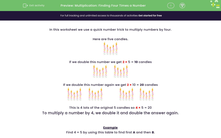# Use double and double again (x4)

In this worksheet, students multiply numbers by 4 by doubling and doubling again.Key stage:  KS 2

Curriculum topic:   Number: Multiplication and Division

Curriculum subtopic:   Write Multiplication/Division Statements for Known Tables

Difficulty level:#### Worksheet Overview

In this activity, we will learn to use a quick number trick to multiply numbers by four.

Learning the four times table is important, but sometimes mental calculations can be useful to find multiplication facts too.

So, we will be using the skills of doubling to help find the answers to questions where we need to multiply by four.

Let's look at an example.

Here are five candles.If we double this number we get 2 × 5 = 10 candlesIf we double this number again we get 2 × 10 = 20 candlesThis is 4 lots of the original 5 candles so 4 × 5 = 20

So, to multiply a number by 4, we double it and double the answer again.

Let's try an example.

Example

Find 4 × 5 by using this table to find first A and then B.

Double 5  is
 A
Double
 A
is
 B
Then 4 × 5  is
 B

Double 5 is 10, so A is 10.

Double 10 is 20, so B is 20.

So 4 × 5 is 20.

Now, we've looked at the method, you are ready to try some questions in the same style.

### What is EdPlace?

We're your National Curriculum aligned online education content provider helping each child succeed in English, maths and science from year 1 to GCSE. With an EdPlace account you’ll be able to track and measure progress, helping each child achieve their best. We build confidence and attainment by personalising each child’s learning at a level that suits them.

Get started